New Foundations Explorer < Previous   Next > Nearby theorems Mirrors  >  Home  >  NFE Home  >  Th. List  >  a9e Unicode version

Theorem a9e 1951
 Description: At least one individual exists. This is not a theorem of free logic, which is sound in empty domains. For such a logic, we would add this theorem as an axiom of set theory (Axiom 0 of [Kunen] p. 10). In the system consisting of ax-5 1557 through ax-14 1714 and ax-17 1616, all axioms other than ax9 1949 are believed to be theorems of free logic, although the system without ax9 1949 is probably not complete in free logic. (Contributed by NM, 5-Aug-1993.)
Assertion
Ref Expression
a9e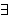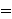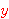Proof of Theorem a9e
StepHypRef Expression
1 ax9 1949 . 2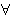2 df-ex 1542 . 2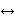31, 2mpbir 200 1Colors of variables: wff setvar class Syntax hints:wn 3wal 1540wex 1541 This theorem was proved from axioms:  ax-1 5  ax-2 6  ax-3 7  ax-mp 8  ax-gen 1546  ax-5 1557  ax-17 1616  ax-9 1654  ax-8 1675  ax-6 1729  ax-7 1734  ax-11 1746  ax-12 1925 This theorem depends on definitions:  df-bi 177  df-an 360  df-tru 1319  df-ex 1542  df-nf 1545 This theorem is referenced by:  equs4  1959  equvini  1987  ax11vALT  2097  axi9  2330  dmi  4919
 Copyright terms: Public domain W3C validator